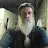APP Information

 Download Version 1.0.36 (13) Apk Size 5.97 MB App Developer DROID FOUNDRY Malware Check TRUSTED Install on Android 4.2.x and up App Package com.droidfoundry.calculator.apk MD5 922cd68fc303727c98ff752e2c4889bb Rate 5### App Description

```Scientific calculator with maths formulas is a best education tool. There are more than 1000 formulas organised neatly. The In-Built calculator has both scientific mode and standard mode. The app also features built in maths related Applications and converters.

Best Features of the App
⋆ Calculator ⋆
• Basic Calculations like addition, subtraction, multiplication and division.
• Advanced scientific calculations like logarithmic, trigonometric, and exponential functions
• comes with Dark theme and light theme with various colours.
• chose between radian and degrees

⋆ Maths Formulas ⋆
• Algebra : Factoring, Roots, Powers, Complex Numbers and Equations.
• Geometry : Cone, Cylinder, Square, Sphere, Rectangle, Triangle, Trapezoid. Etc…
• Analytical Geometry : Circle, Hyperbola, Ellipse, Parabola etc…
• Integration Functions : Properties of Integration, rational functions, Trigonometric Functions, Hyperbolic functions, Exponential and log functions
• Derivations : Properties of derivation, general derivative, hyperbolic and inverse hyperbolic functions etc…
• Trigonometry : Sine and cosine Rule, Table of angle, Angle transformations, Euler's formula etc…
• Laplace Transformation : Properties and Functions of Laplace transformation
• Numerical Methods : Roots of Equation, Numerical Integration, Lagrange and Newton's Interpolation
• Probability : Expectation, Variance, Distribution, Permutation and Combination etc…
• Fourier Series : Fourier transform operations and Table of Fourier Transform
• Series : Arithmetic Series, Geometric Series, Finite and Binomial Series.
• Formulas Search Functionality
• Matrices : Transpose, Addition, Multiplication, Inverse of a Matrix
• Equations : Linear, Quadratic, Exponential, Log Equations and InEquations
• Surface Area, 3D - Geometry, Coordinated Geometry, Logarithms, Circles, Planes

⋆ Maths Applications ⋆
• Number Base Converter : Binary, Decimal, Octal and HexaDecimal Converters
• Number Series Generator : Arithmetic Series, Geometric Series, Fibonacci Series
• Volume Calculation : Volume of Cone, Cylinder, Rectangle, Circle, Tent and Trapezoid
• Area Calculation : Area of Circle, Rectangle, Triangle and Trapezoid.
• Roman Numerals : Decimal Number to Roman Numeral Converter.
• Ratio Calculator
• Proportion Calculator
• Decimal to Fraction Converter
• GCD and LCM calculator
• Random Number Generator

P.S Maths Formulas with Calculator is created proactively with wide range of users from Students, Engineer and Professionals in mind. This app is a irreplaceable daily utility for those who find it difficult to keep track of all important maths and Engineering Formulas. The in-built Scientific Calculator and Maths Applications and Converter is a added bonus feature of the App. Thanks for your continuous Support. We have lot more formulas and features coming up in the future updates.
```

### App ChangeLog

• Version 1.0.36
• ✓ Maths Application, Calculator, Surface area, Perimeter
• ✓ Improved user experience
• ✓ 1000+ Physics Formulas and concepts : Velocity, Gravity, Frequency, Acceleration and more
• ✓ Chemistry Formulas : Atom, Mole, Gas Law, Solubility and more
• ✓ Minor fixes and enhancements

### More Android Apps to Consider

1.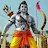Red Leaf

Very useful app for every person who interested in math and formula and trigonometry .in real this app is very good but remove ads

2.this will help solving math problems for my kids using modular

3.Sai Kumar

Ilike this app so much because all formulas reference

4.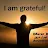Jamshid Elham

It should be more clear than this that every one can use this

5.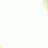Reallly ohh this is an application that really helps me do math problems

6.akash chandra

best app ever seen good for all student thanks for making app

7.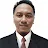Almaududi -

Good applications... Thanks for this..

8.Parniyan Sh

just have a simple calculator

9.10.